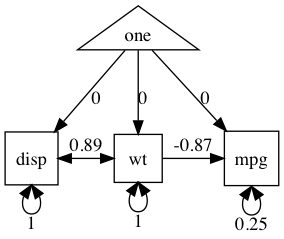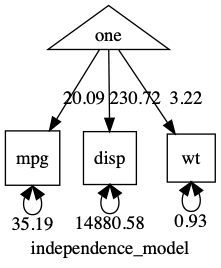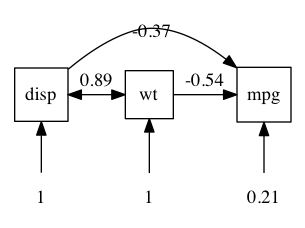# umx!

## Build & run a model in a minute!

umx stands for “user” OpenMx helper library. It’s purpose is to help with Structural Equation Modeling in R.

I called this post “first steps” but it will take you a long way into practical modeling. So, let’s do some modeling …

If you haven’t installed umx, do that now, and follow the link back here.

``````# load umx library
library("umx")
``````

note: umx help is not just boilerplate documentation: All functions have real-world examples and tips!.

## Overview

For those of you who like to get straight to the code: here’s what happens on this page:

``````m2 = umxRAM("big and heavy", data = mtcars,
# One headed paths from disp and weight to mpg
umxPath(c("disp", "wt"), to = "mpg"),
# Allow predictors to Covary
umxPath("disp", with = "wt"),
# free variances and means for each manifest
umxPath(v.m. = c("disp", "wt", "mpg"))
)

umxSummary(m2, std = TRUE)

# Update m2 by dropping mpg ~ displacement, which has label "disp_to_mpg"
m3 = umxModify(m2, update = "disp_to_mpg", name = "drop effect of capacity", comparison = TRUE)

``````

umxSummary table of model 2

name Std.Estimate Std.SE CI
disp_to_mpg -0.36 0.18 -0.36 [-0.71, -0.02]
wt_to_mpg -0.54 0.17 -0.54 [-0.89, -0.2]
mpg_with_mpg 0.22 0.07 0.22 [0.08, 0.35]
disp_with_disp 1.00 0.00 1 [1, 1]
disp_with_wt 0.89 0.04 0.89 [0.81, 0.96]
wt_with_wt 1.00 0.00 1 [1, 1]

χ²(87) = 0, p < 0.001; CFI = 1; TLI = 1; RMSEA = 0

umxCompare model 2 and model 3

Model EP Δ -2LL Δ df p AIC Compare with Model
big and heavy 9       419.1183
drop effect of capacity 8 3.8616447 1 0.049 420.9800 big and heavy
``````plot(m4)
``````Now, let’s build, run, summarize, modify/compare, and display this model step by step.

### Two theories to compare

Science consists of making and comparing different theoretical predictions: the idea that there are, say, two forms of dyslexia is tested not against a null hypothesis that there are none, but against some competing model: that there is one form, for instance, or perhaps a model that reading disorder is just a problem with vision.

Whereas the answer to the question “does this theory fit better than no theory” is quasi-always “yes (if we have enough subjects)”, that’s an incredibly low bar. It also doesn’t lead to increases in knowledge: these come from pitting models (theories generated by human creativity) against each another, to see which is closer to the truth.

This bed-rock metric of closer-further gives us a boot strap to iteratively choose among ideas that are ever closer to reality. It is captured in likelihood differences, which we will use here to test our competing models.

Here, we begin with two patsy ideas: Theory one: miles/gallon (mpg) goes down linearly with increases in car mass car and as engine size (capacity) goes up. Our contrasting theory predicts that “only weight matters”, not engine capacity. We can compare the two models by building model 1, then dropping capacity and testing the idea that the only determinant (in this confounded play dataset) of mpg is weight (in which case fit should not reduce significantly).

We will use the built-in mtcars data set. miles/gallon is `mpg`, displacement is `disp`, and weight is `wt`.

note: `mtcars` + Henderson & Velleman’s (1981) “Building Multiple Regression Models Interactively” can teach you a lot of statistics in a very short period of time: Recommended!

We’ll use `umx` to build both models, and compare them.

### Building on what you already know

In `lm`, model 1 would be `mpg ~ disp + wt`. Model 2 would be `mpg ~ disp`

Sewall Wright invented SEM to allow us to think in explicit graphs. So, here’s what that language implies: in the figure “A model of Miles/gallon”

Let’s start off with something very simple: the means and variances of three raw variables. This is also called an “independence model”.

The `umx` equivalent of `lm` is `umxRAM`, and we build the “formula” using `umxPath`s. here’s the whole model:

``````manifests = c("disp", "wt", "mpg")
m1 <- umxRAM("my_first_model", data = mtcars,
umxPath(var   = manifests),
umxPath(means = manifests)
)
``````

These two steps of giving a variable a variance and a mean are so common, umx has a shortcut “v.m.”

``````m1 = umxRAM("independence_model", data = mtcars,
umxPath(v.m. = c("disp", "wt", "mpg"))
)
``````

Like `lm`, we’re going to feed this model-container a data set (`data = mtcars`). The string “independence_model” is a name that is used to refer to the model, and which is used in output as well (so make it informative).

We then give `umxRAM` a list of `umxPaths` to specify all the lines, boxes, and circles in the figure above.

With `umxPath`, you can specify a variance (a 2-headed path originating and terminating on one variable) with the argument `var =` To specify a mean (a path from the constant one to a variable), just use the argument `means =`. You can learn more about umxPath in the help and in this chapter on using umxPath.

By default, just like `lm`, `umxRAM` runs the model automatically for you. It also prints out a table of fit-information.

nb: you can re-run a model anytime with `mxRun`

You can also request a summary, and plot the output:

``````umxSummary(m1)
plot(m1)
``````

Here’s the plot:note: When you are running real models, having variances differ by orders of magnitude can make it hard for the optimizer. In such cases, you can often get better results making variables more comparable: in this case, for instance, by converting displacement into litres to keep its variance closer to that of the other variables. (see here for a post on renaming variables (like “disp” to “displacement”), and scaling variables/data-wrangling)

As you can see, this is an “independence model”: No covariances were included, so all variables are modeled as uncorrelated. It would fit poorly in this case. `umxSummary` tells us this fit can definitely be improved: χ²(90) = 98.32, p < 0.001; CFI = 0; TLI = 0; RMSEA = 0.996

Clearly some un-modeled covariance here… Let’s build our theorized model.

``````m2 <- umxRAM("big and heavy", data = mtcars,
# One headed paths from disp and weight to mpg
umxPath(c("disp", "wt"), to = "mpg"),
# Allow predictors to Covary
umxPath(cov = c("disp", "wt")),
# Variances and Means
umxPath(means = c("disp", "wt", "mpg")),
umxPath(var = c("disp", "wt", "mpg"))
)
``````

nb: remember: the shortcut for the last two lines is: `umxPath(v.m. = c("disp", "wt", "mpg"))`

You can compare the models with

``````umxCompare(m2, m1)
``````

This new model is better, i.e., the three degrees of freedom were worth paying for in improved fit to the data:

Model EP Δ -2LL Δ df p AIC Compare with Model
1 big and heavy 9 419.12
2 independence_model 6 98.312 3 < 0.001 511.44 big and heavy

In fact this (saturated) model fits perfectly, as `umxSummary` shows: χ²(87) = 0, p < 0.001; CFI = 1; TLI = 1; RMSEA = 0

We can request a full summary including standardized output as a table with (“show = std” requests the standardized paths):

``````umxSummary(m2, std = TRUE)
``````
name Estimate Std.Error CI (SE-based)
1 disp_to_mpg -0.37 1.8e-01 -0.37 [-0.72, -0.02]
2 wt_to_mpg -0.54 1.8e-01 -0.54 [-0.89, -0.2]
3 mpg_with_mpg 0.21 6.8e-02 0.21 [0.08, 0.35]
4 disp_with_disp 1.00 1.9e-12 1 [1, 1]
5 disp_with_wt 0.89 3.7e-02 0.89 [0.82, 0.96]
6 wt_with_wt 1.00 2.5e-13 1 [1, 1]

We can plot these standardized (or raw) coefficients on a diagram the way Sewall Wright would like us too:

``````plot(m2,  means = FALSE) # plot has lots of control: here we suppress display of the means
``````note: Means are not shown on this diagram (`showMeans =FALSE`) though they are in the model.

We can ask for the (unstandardized) confidence intervals with the usual `confint` function. Because these can take a long time for SEM models, the default is to require you to ask to run them.

``````    confint(m2, run = TRUE)
``````
lbound estimate ubound
disp_to_mpg -0.035 -0.018 0.000
wt_to_mpg -5.641 -3.351 -1.060
mpg_with_mpg 4.866 7.709 13.642
disp_with_disp 4536 15360 24081337
disp_with_wt 58.605 107.685 286.965
wt_with_wt 0.589 0.951 1.590
one_to_mpg 30.631 34.960 39.291
one_to_disp 173.627 230.815 287.822
one_to_wt 2.871 3.218 3.560

What did lm think these should be?

``````l1 = lm(mpg ~ 1 + disp + wt, data = mtcars)
coef(l1)
``````
(Intercept) disp cyl
34.96055404 -0.01772474 -3.35082533
``````    confint(l1)
``````
2.5 % 97.5%
(Intercept) 30.53357368 39.387534392
disp -0.03652128 0.001071794
wt -5.73173459 -0.969916079

Next, we can modify and compare this model, with one in which only weight affects mpg.

## Modify and Compare models: The secret-sauce of science

Fundamentally, modeling is in the service of understanding causality and we do that primarily via model comparison: Better theories fit the data better than do worse theories.

So, we can ask questions like “does lower weight give better miles per gallon”?

In graph terms, this is asking, “can I set the path from to wt to mpg to zero without significant loss of fit?”

There are two ways to test this with umx.

First, we can modify m2 by overwriting the existing path with one fixing the value to zero.

With umxPath we can save some typing and use `fixedAt`

``````m3 = umxRAM(m2, umxPath("disp", to = "mpg", fixedAt = 0), name = "weight_doesnt_matter")
``````

As you develop skill with umx, you ‘ll often umxModify a model, instead of building a whole new model

``````m3 = umxModify(m2, update = "disp_to_mpg", name = "weight_doesnt_matter")
``````

That examines our competing theoretical prediction, with a “zero” path from `wt` to `mpg` (weight of car to fuel economy)

## Compare two models

Now we can test if weight affects mpg by comparing these two models:

``````umxCompare(m2, m3)
``````

As you develop skill with umx, you might umxModify and compare in one step:

``````m3 = umxModify(m2, update = "disp_to_mpg", name = "weight_doesnt_matter", comparison = TRUE)
``````

The table below shows that dropping this path caused a (just) significant loss of fit (χ²(1) = 3.96, p = 0.049):

Model EP Δ -2LL Δ df p AIC Compare with Model
big and heavy 9 419.13     419.12
weight_doesnt_matter 8 3.86 1 0.049 420.98 big and heavy

The AIC moved the wrong direction, p-value is marginal. This model would lead us to conclude that weight matters, but only a little bit (controlling for engine capacity). Note however that these variables covary: heavy vehicles have big motors: This is why trying to do science on observational data is fraught with problems. MUCH better to systematically vary the weight in a randomized controlled trial and measure mpg change. In behavior science, Twin Studies and Mendelian Randomization let us do this.

Advanced tip: `umxModify()` can modify, run, and compare all in 1-line!

For instance to drop the path from wt to mpg, we can say:

``````m4 = umxModify(m2, update = "wt_to_mpg", name = "drop effect of wt", comparison = TRUE)
``````

You will use `umxModify` often.

By default, `umxModify` fixes the value of matched labels to zero. Learn more at the umxModify tutorial.

tip: To discover the labels in a model, use `parameters(model)` (or just call `umxModify` with no update)

The `umx` version of `parameters` is on steroids - you can filter using wild card patterns! So

``````umxGetParameters(m3, pattern = "^mpg")
#  "mpg_to_mpg"   "mpg_to_disp"  "mpg_to_wt"    "mpg_with_mpg" "mpg_with_wt"
``````